# I.E. Irodov Solutions on Properties of Atoms. Spectra

Atoms are the smallest unit of matter. It acts as building blocks for everything. Atoms have various properties and are of great importance. Similarly, Properties of Atoms Spectra play a vital role in Physics. As the name implies, this chapter deals with the properties of atomic spectra. IE IRODOV Physics Solutions provides the solutions to various problems related to the concept. The questions are designed in such a manner that they cover the vital concepts and give detailed explanations to all the problems.

The solutions are based on the various formulae, equations and diagrams which come under this topic. It helps the students understand the concept and connects the theoretical concepts with practical problems. Students can easily understand and have a grip over the chapter. Students can easily face the upcoming board and competitive examination on solving and practising the problems. This helps in better understanding and building confidence in students.

### I.E. Irodov Solutions on Properties of Atoms. Spectra

1. The binding energy of a valence electron in a Li atom in the states 2S and 2P is equal to 5.39 and 3.54 eV respectively. Find the Rydberg corrections for S and P terms of the atom.

Solution:

1. From the Rydberg formula we know that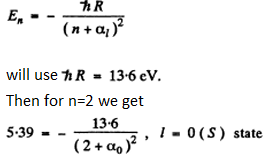α1 = -0.41

For p state

3.54=-\frac{13.6}{(2+\alpha _{1})^{2}}

α1 = -0.039

2. Find the Rydberg correction for the 3P term of a Na atom whose first excitation potential is 2.10 V and whose valence electron in the normal 3S state has the binding energy 5.14 eV.

Solution:

1. The energy of the 3p state must be -(E0-eϕ) where - E0 is the energy of the 3S state.

Then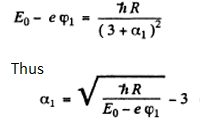3. Find the binding energy of a valence electron in the ground state of a Li atom if the wavelength of the first line of the sharp series is known to be equal to λ1 = 813 nm and the short-wave cutoff wavelength of that series to λ2 = 350 nm.

Solution:

1. For the first line of the sharp series (3S→2P) in a Li atom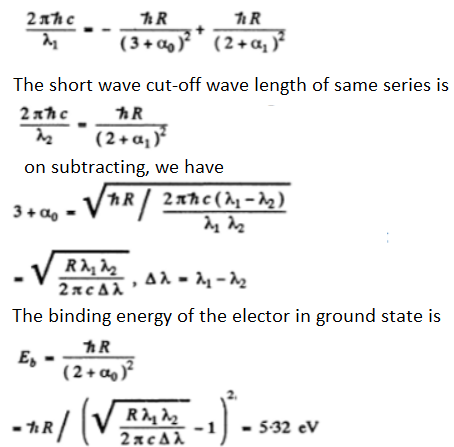4. Determine the wavelengths of spectral lines appearing on transition of excited Li atoms from the state 3S down to the ground state 2S. The Rydberg corrections for the S and P terms are —0.41 and —0.04.

Solution:

1.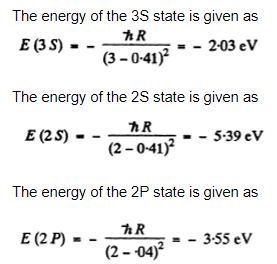We see that

E (2S ) <E (2P)< E ( 3 S)

The transitions are 3S → 2P and 2P →2S.

Direct 3S → 2S transition is forbidden by selection rules. The wavelengths are determined by

E_{2}-E_{1}=\Delta E=\frac{2\pi hc}{\lambda }

On substitution we get

λ = 0.816 μm ( 3S → 2P )

and λ = 0.674 μm ( 2P → 2S )

5. The wavelengths of the yellow doublet components of the resonance Na line caused by the transition 3P → 3S are equal to 589.00 and 589.56 nm. Find the splitting of the 3P term in eV units.

Solution:

1. The splitting of the Na lines is due to the fine structure splitting of 3 p lines (The 3 s state is nearly single except for possible hyperfine effects.) The splitting of the 3 p level then equals the energy difference

\Delta E=\frac{2\pi hc}{\lambda_{1} }-\frac{2\pi hc}{\lambda_{2}}=\frac{2\pi hc(\lambda _{2}-\lambda _{1})}{\lambda _{2}\lambda _{1}}=\frac{2\pi hc\Delta \lambda }{\lambda^{2} }

Here Δλ = wavelength difference & λ = average wavelength.

On substitution we get

ΔE = 2.0 meV

6. The first line of the sharp series of atomic cesium is a doublet with wavelengths 1358.8 and 1469.5 nm. Find the frequency intervals (in rad/s units) between the components of the sequent lines of that series.

Solution:

1. The sharp series arise from the transitions ns → mp . The s lines are unsplit so the splitting is due entirely to the p level. The frequency difference between sequential lines is \frac{\Delta E}{h} and is the same for all lines of the sharp series. It is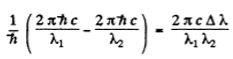On evaluating we get

7. Write the spectral designations of the terms of the hydrogen atom whose electron is in the state with principal quantum number n = 3.

Solution:

1. We shall ignore hyperfine interaction. The state with principal quantum number n = 3 has orbital angular momentum quantum number / = 0, 1,2

The levels with these terms are 3 5, 3 P, 3 D. The total angular momentum is obtained by combining spin and angular momentum. For a single electron, this leads to

J=½ if L=0

J=L-½ and L+½ if L≠0

We then get the final designations like

3S1/2, 3P1/2, 3P3/2, 3D3/2, 3D5/2

8. How many and which values of the quantum number J can an atom possess in the state with quantum numbers S and L equal respectively to

(a) 2 and 3;

(b) 3 and 3;

(c) 5/2 and 2?

Solution:

1. The rule is that if \vec{J}=\vec{L}+\vec{S} then J takes the value |L-S| to L+S

(a) The values are 1, 2, 3, 4, 5

(b) The values are 0, 1, 2, 3, 4, 5, 6

(c) The values are ½, 3/2, 5/2, 7/2, 9/2

9. Find the possible values of total angular momenta of atoms in the states 4P and 6D.

Solution:

1. For the state 4 p, L = 1, S = 3/2,(since 2s + 1 = 4 ) . For the state 5 d, Z, = 2 , s = 2 The possible values of J are

J:5/2, 3/2,1/2 for 4p

The value of the magnitude of angular momentum is h√J(J+1)

On substituting the values, we get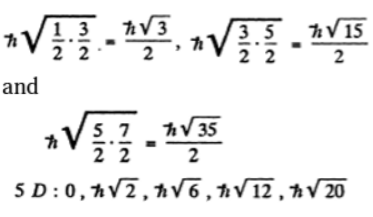10. Find the greatest possible total angular momentum and the corresponding spectral designation of the term

(a) of a Na atom whose valence electron possesses the principal quantum number n = 4; (b) of an atom with electronic configuration 1s22p3d.

Solution:

1. (a) For the Na atoms the valence electron has principal quantum number n = 4, and the possible values of orbital angular momentum are l = 0 , 1, 2 , 3 so lmax = 3 . The state is 2F, maximum value of J is 7/2

Thus the state with maximum angular momentum will be

For this state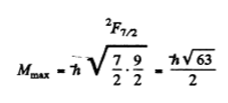(b) For the atom with electronic configuration 1 s22p 3d .There are two inequivalent valence electrons. The total orbital angular moments will be 1, 2, 3 so we pick l = 3. The total spin angular momentum will be s = 0, 1 so we pick up s = 1. Finally, 7 will be 2, 3, 4 so we pick up 4. Thus maximum angular momentum state for this state is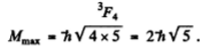11. It is known that in F and D states the number of possible values of the quantum number J is the same and equal to five. Find the spin angular momentum in these states.

Solution:

1. For the f state L = 3, For the d state L = 2. Now if the state has spin s the possible angular momentum are |L-S| to L+S.

The number of J angular momentum values is 2 S + 1 if L ≥ S and 2 L + 1 if L < S.

Since the number of states is 5, we must have S ≥ L = 2 for D state while S ≤ 3 and 2 S + 1 = 5 in ply S = 2 for F state.

Thus for the F state total spin angular momentum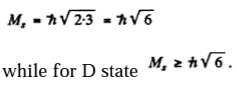12. An atom is in the state whose multiplicity is three and the total angular momentum is {\not{h}}\sqrt{20}. What can the corresponding quantum number L be equal to?

Solution:

1. Multiplicity is 2S + l so S = 1

Total angular momentum is {\not{h}}\sqrt{J(J+1)} so J = 4. Then L must equal 3,4,5.

In order that J = 4 may be included in

|L-S| to L+S.

13. Find the possible multiplicities x of the terms of the types

(a) xD2

(b) xHP3/2

(c) xF1

Solution:

1. (a) Here J = 2, L = 2. Then S = 0, 1 , 2, 3, 4 and the multiplicities( 2S - 1 ) are 1, 3, 5, 7, 9.

(b) Here J = 3 / 2 , L = 1 Then S= 5/2, 3/2, ½ and the multiplicities are 6, 4, 2

(c) Here J = 1, L = 3. Then S = 2 , 3 , 4 and the multiplicities are 5, 7, 9

14. A certain atom has three electrons (s, p, and d), in addition to filled shells, and is in a state with the greatest possible total mechanical moment for a given configuration. In the corresponding vector model of the atom find the angle between the spin momentum and the total angular momentum of the given atom.

Solution:

1. The total angular momentum is greatest when L, S are both greatest and add to form J. Now for a triplet of s, p> d electrons Maximum spin→S =3/2 corresponding to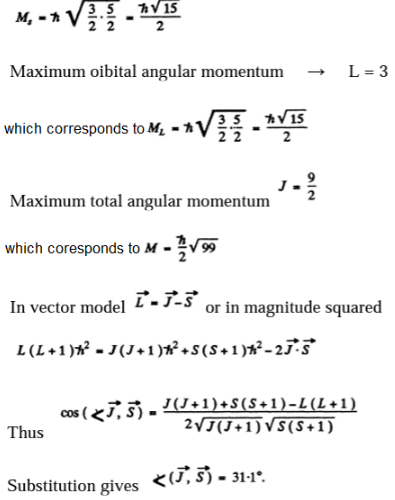15. An atom possessing the total angular momentum {\not{h}}\sqrt{6} is in the state with spin quantum number S = 1. In the corresponding vector model, the angle between the spin momentum and the total angular momentum is θ = 73.2°. Write the spectral symbol for the term of that state.

Solution:

1. Total angular momentum {\not{h}}\sqrt{6} means J = 2. It is gives that S = 1. This means that L = 1,2, or 3 . From vector model relation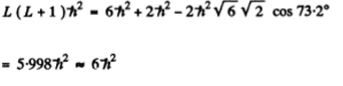Thus E = 2 and the spectral symbol of the state is 3D2

16. Write the spectral symbols for the terms of a two-electron system consisting of one p electron and one d electron.

Solution:

1. In a system containing a p electron and a d electron S = 0,1 L = 1,2,3

For S = 0 we have the terms

1P1, 1D2, 1F3

For S = 1 we have the terms

3P0 , 3P1, 3P2, 3D1, 3D2, 3D3, 3F1, 3F2, 3F3

17. A system comprises an atom in 2P3/2 state and a d electron. Find the possible spectral terms of that system.

Solution:

1. $1= ½, l1=1, j = 3/2 The electron has$2= ½, l2=2 so the total angular momentum quantum number must j2=3/2 or 5/2.

In L - S compiling we get S = 0, 1 . L = 1,2, 3 and the terms that can be formed are the same as written in the problem above. The possible values of angular momentum are consistent with the addition j1= 3/2 0r j2= 3/2 or 5/2.

The latter gives us J= o, 1, 2, 3; 1,2,3,4

All these values are reached above

18. Find out which of the following transitions are forbidden by the selection rules:

2D3/22P1/2, 3P12S1/2, 3F33P2, 4F7/24D5/2

Solution:

1. Selection rules are ΔS = 0

\Delta L=\pm 1

\Delta J=0, \pm 1 (as no 0→0)

Thus,

2D3/22P1/2 is allowed

3P12S1/2 is allowed

3F33P2 is not allowed

4F7/24D5/2 is allowed

19. Determine the overall degeneracy of a 3D state of a Li atom. What is the physical meaning of that value?

Solution:

1. For a 3 d state of a Li atom, S= ½ because there is only one electron and L = 2

The total degeneracy is g= (2L + 1) (2S + 1) = 5 x 2 = 10.

The states are 2D3/2 and 2D5/2. and we check that

g = 4 + 6 = {2 X 3/2+1} {2 X 3/2+1}

20. Find the degeneracy of the states 2P, 3D, and 4F possessing the greatest possible values of the total angular momentum.

Solution:

1. The state with greatest possible total angular momentum are

For

2P state

J= ½ + 1 = 3/2 i.e 2P3/2

Its degeneracy is 4.

For

3D state

J= 1 + 2 = 3 i.e 3D3

Its degeneracy is 2 x 3 + 1=7

For

4F state

J= 3/2 + 3 = p/2 i.e 4F4/2

Its degeneracy is 2 x 9/2 + 1=10.